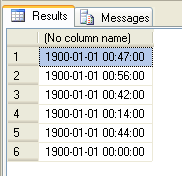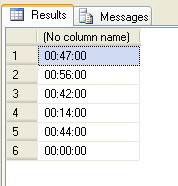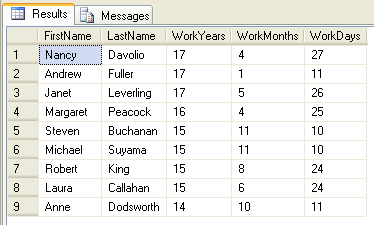Web www.beansoftware.com
Home

# How To Use T-SQL To Subtract Dates And Calculate Time Span?

From time to time, you'll need to find out duration of some process, get age from birthday, find how many days till anniversary, etc. In cases like this, you need to subtract two dates and calculate difference (time span) between them.

The simplest approach is to subtract times directly. If you try:

SELECT FinishTime - StartTime FROM WorkLog

Result will be something like this:As you see, my example table contains small time spans in minutes. Resulted data type is like original columns, smalldatetime in my case. Time span in minutes is calculated correctly but the year is 1900 which is incorrect. Year 0 would logically be expected as correct result. I got year 1900 because earliest date for datetime or smalldatetime data types is 1/1/1900 and earlier years are not accepted. Although final result is not time span but datetime from 18th century, this way can be very efficient in some scenarios, like common example when you need to format short time spans as "hh:mm:ss".

## How to format duration between two datetime or smalldatetime as hh:mm:ss

There are many examples on Internet about how to format durations between datetime columns and many of them use string manipulation to get acceptable result. String manipulation is always considered as slow method and should be avoided if it is possible. Instead of large procedure or function and using of RTRIM, RIGHT, CASE and who knows what more, to subtract two dates and format output duration as HH:mm:ss use this single line of SQL:

SELECT CONVERT(varchar,(FinishTime - StartTime), 108) FROM WorkLog

Results will be time spans correctly formatted in hh:mm:ssIf you want to include milliseconds too, just set third parameter to 104, instead of 108:

SELECT CONVERT(varchar,(FinishTime - StartTime), 104) FROM WorkLog

You can't use this approach to get completely correct time periods that includes years or months. But, it is possible to try to correct year (subtract 1900), month and day (subtract 1) and get almost perfect solution. For example, let say you want know how long every employee works in a company. If SQL Server example database Northwind is used, example could look like this:

USE Northwind
GO

SELECT
FirstName,
LastName,
-- Correct number of years
(YEAR(WorkDuration) - 1900) AS WorkYears,
-- Correct months
(MONTH(WorkDuration) - 1) AS WorkMonths,
-- Correct days
(DAY(WorkDuration) - 1) AS WorkDays
FROM
(SELECT
FirstName, LastName, (GETDATE() - HireDate) AS WorkDuration
FROM
Employees) tmp

This will produce output with names of employees and year, month and days worked. We can include hours, minutes, seconds and even milliseconds but this usually is not necessary in this case. Output will look like in image bellow:Note, all years and months are not the same. Some year has 365 days, other 366 days. February has 28 or 29 days, January has 31 days, April 30 days etc. Because of this, calculated result will probably have small deviation from real value. For example, 31 days means 1 month and 1 day if that month is April, but means 1 month and 0 days if month is January. If total accuracy is not demanded, then you can consider this method since it is very simple.

## How to get precise time difference between two datetime or smalldatetime columns

As you see, direct subtraction of dates can be useful in some scenarios but it is very limited. To get time difference between datetime or smalldatetime columns you can use DATEDIFF function. For example, to find duration in days between two dates you can use this code:

SELECT DATEDIFF(d, StartTime, FinishTime)

As you see DATEDIFF function has three parameters. First parameter specifies measurement unit. You can use these values:

Datepart Abbreviation
year yy, yyyy
quarter qq, q
month mm, m
dayofyear dy, y
day dd, d
week wk, ww
hour hh
minute mi, n
second ss, s
millisecond ms

Second parameter is start date. This could be smalldatetime or datetime data type or string that is formatted as a date.

Third parameter is ending date of time span. Note, if smalldatetime is used, seconds and milliseconds will always be zero.

DATEDIFF function returns integer value. For example, if you try to find out how much hours are in 61 minutes DATEDIFF will return that there are 2 hours. You can be satisfied with this or try to get more precise value with additional calculation. Just use smaller time unit to calculate bigger. In this example we could find number of seconds first and then divide it with 3600. It is pretty simple to do this for minutes, hours and days. But when you come to months and years, there is again questions would you consider month as a 28, 29, 30 or 31 days, is year 365 or 366 or maybe is better 365.25 days etc.

### DATEDIFF function precaution

Since DATEDIFF returns an integer values, you should be careful when you use small time units (e.g. milliseconds) on large time periods. Because of this, DATEDIFF will return an out of range error if value is larger than maximum for int data type. To avoid these errors, remember that for milliseconds that value is a little more of 24 days and 20 hours, and maximum time span for seconds is about 64 years.

Related articles:

1. SQL Server Optimization For ASP.Net Developer

FAQ toolbar: Submit FAQ  |  Tell A Friend  |  Add to favorites  |  Feedback